# Introduction

This is a lesson introducing you to making plots with the R package ggplot2. The ggplot2 package was originally developed by Hadley Wickham and is now developed and maintained by a huge team of data visualization experts. It’s an elegant and powerful way of visualizing your data and works great for everything from quick exploratory plots to carefully formatted publication-quality graphics.

Students should already have a beginner-level knowledge of R, including basic knowledge of functions and syntax, and awareness of how data frames in R work.

## Learning objectives

At the end of this course, you will know …

• what the “grammar of graphics” is and how ggplot2 uses it
• how to map a variable in a data frame to a graphical element in a plot using `aes`
• how to use different `geom`s to make scatterplots, boxplots, histograms, density plots, and barplots
• how to add trendlines to a plot
• how to compute summary statistics and plot them with `stat` functions
• how to make plots for different subsets of your data using `facet`s
• how to change the style and appearance of your plots

## What is the grammar of graphics?

The theory underlying ggplot2 is the “grammar of graphics.” This concept was originally introduced by Leland Wilkinson in a landmark book. It’s a formal way of mapping variables in a dataset to graphical elements of a plot. For example, you might have a dataset with age, weight, and sex of many individuals. You could make a scatterplot where the age variable in the data maps to the x axis of the dataset, the weight variable maps to the y axis, and the sex variable maps to the color of the points.

In the grammar of graphics, a plot is built in a modular way. We start with data, map variables to visual elements called `geom`s, and then optionally modify the coordinate system and scales like axes and color gradients. We can also modify the visual appearance of the plot in ways that don’t map back to the data, but just make the plot look better.

If that doesn’t make sense to you, read on to see how this is implemented in ggplot2.

## How does a ggplot work?

These images are taken from the ggplot2 cheatsheet. I recommend downloading this cheatsheet and keeping it handy – it’s a great reference!

ggplot2 uses the grammar of graphics to build up all plots from the same set of building blocks. You specify which variables in the data correspond to which visual properties (aesthetics) of the things that are being plotted (geoms).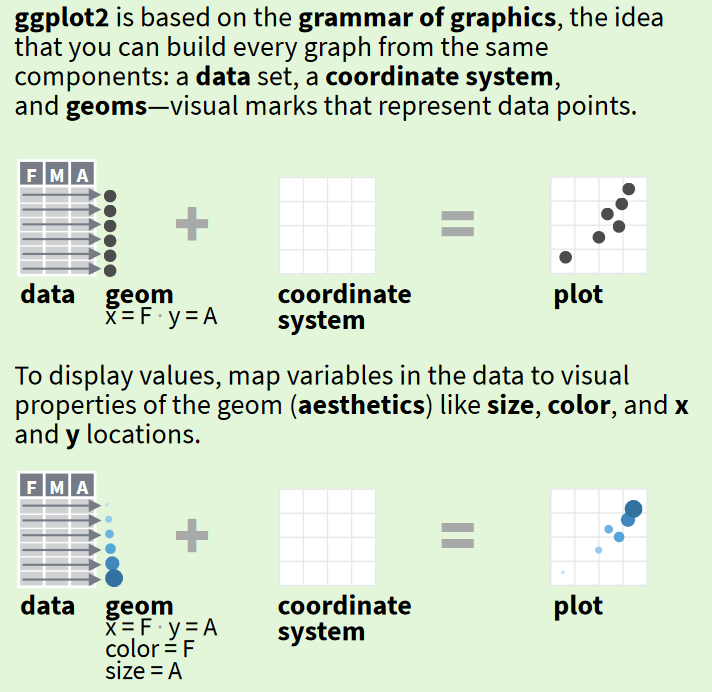In practice, that looks like this: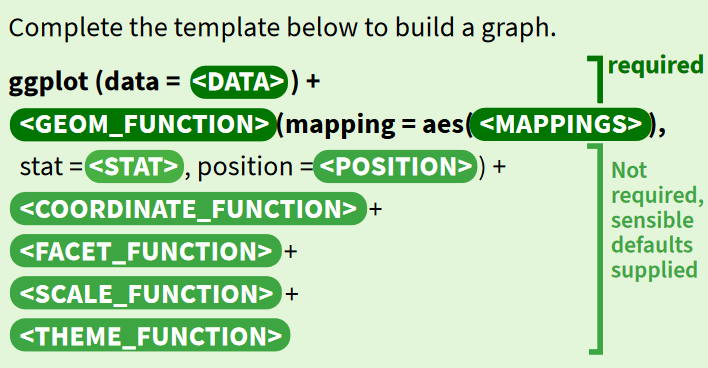As you can see we at least need data, a mapping of variables to visual properties (called `aes`), and one or more `geom` layers. Optionally, we can add coordinate system transformations, scale transformations, facets to split the plot into groups, and themes to change the plot appearance. We will cover all of this (other than coordinate transformation) in this intro lesson.

## The data

In this lesson we’ll use three fun datasets from Kaggle, a data science competition site where users upload public domain datasets. Click on each link if you want to learn more about each dataset, including descriptions of each column.

# The code

We will use only the ggplot2 package in this tutorial. Use the `read.csv()` function from base R to read in each of the three datasets from the URL where they are hosted on GitHub.

``````library(ggplot2)

You can use the `head()`, `summary()`, or `str()` functions to examine each dataset.

## Our first ggplot

Let’s start with a simple scatterplot. Does money buy happiness? We will find out. The `WHR` dataset has a row for each country. We will make a scatterplot with plotting GDP per capita on the x axis and happiness score on the y axis.

Start by calling the `ggplot()` function with the `data` argument saying which data frame contains the plotting data.

``ggplot(data = WHR)``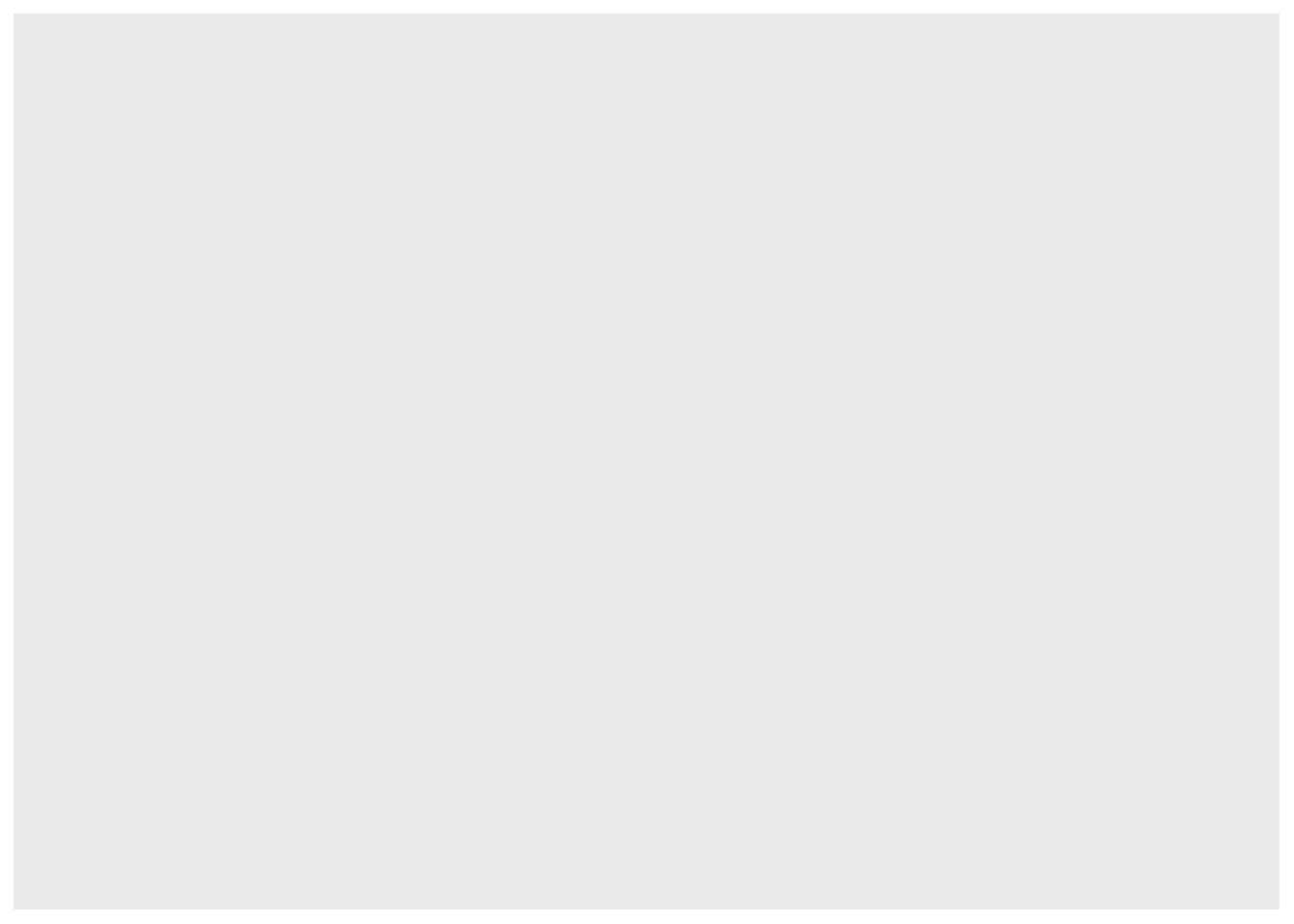That didn’t do anything so far. We’ve only specified the dataset to get the plotting data from, without saying which columns of the dataset will be mapped to which graphical elements.

``ggplot(data = WHR, aes(x = GDP.per.Capita, y = Happiness.Score))``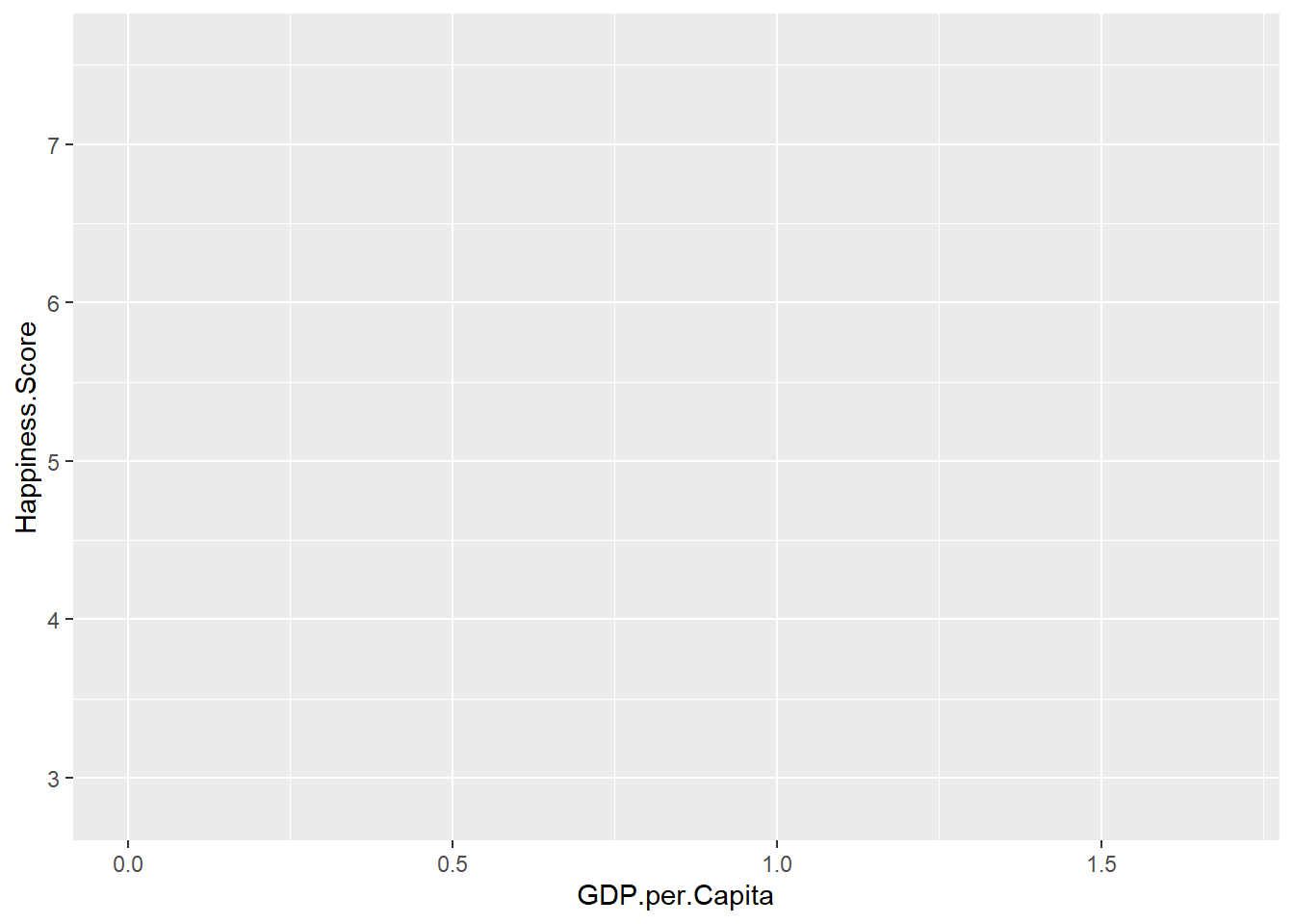Once we add the x and y mappings, we now can see the two axes and coordinate system, which is already set to the range of each variable, but no data yet. We haven’t added any `geom` layers.

``ggplot(data = WHR, aes(x = GDP.per.Capita, y = Happiness.Score)) + geom_point()``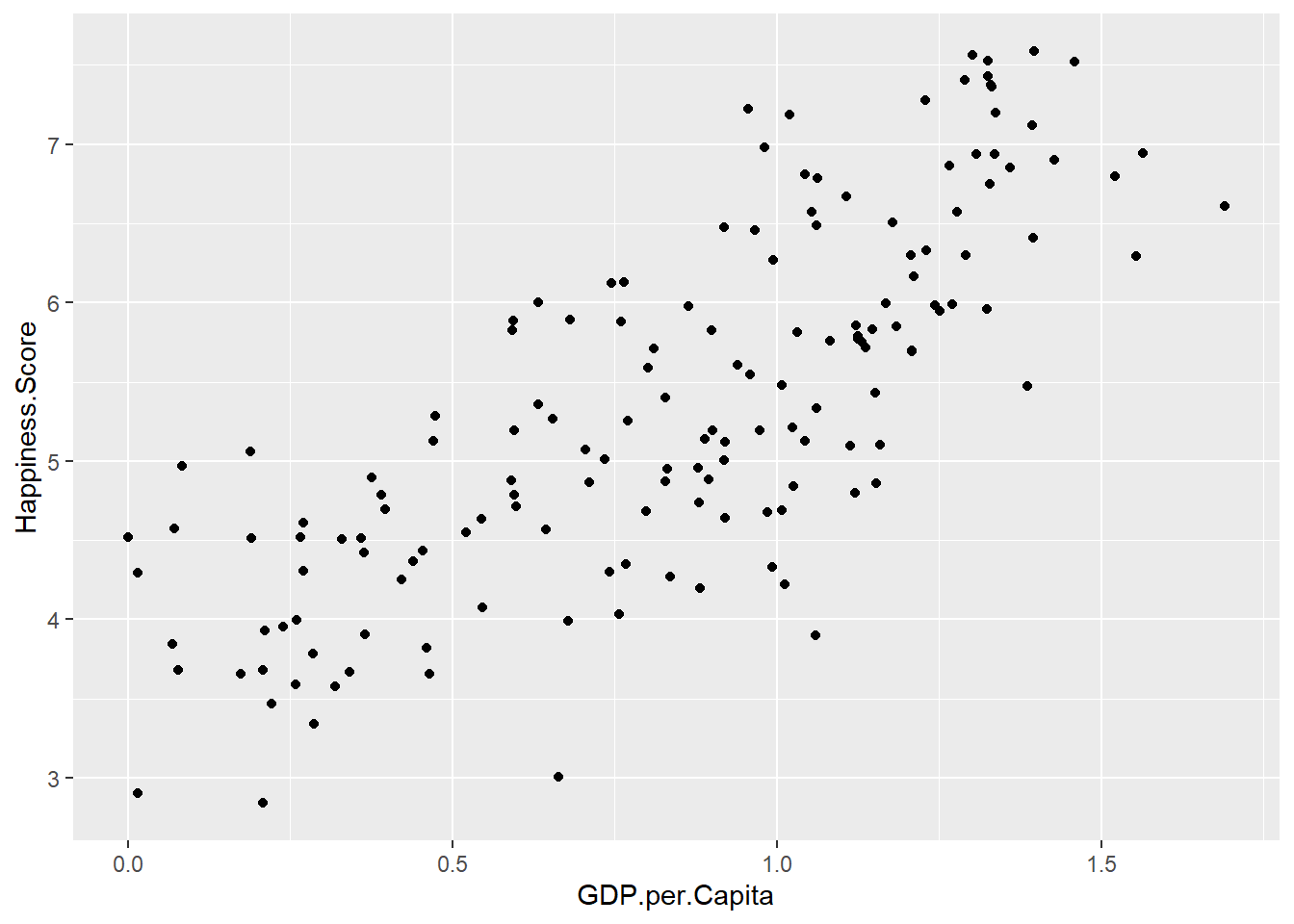By adding a `geom_point()` layer to the plotting code, we have now made a scatterplot!

Notice we use `+` to add each new piece of the plotting code.

### Modifying the plot: changing the geom

We can modify the plot in many ways. One way is by changing the `geom`. This will plot the same data but using a different type of plot. For example we might want to connect data points with lines instead of drawing them as separate points. For that we will replace `geom_point()` with `geom_line()`.

``ggplot(data = WHR, aes(x = GDP.per.Capita, y = Happiness.Score)) + geom_line()``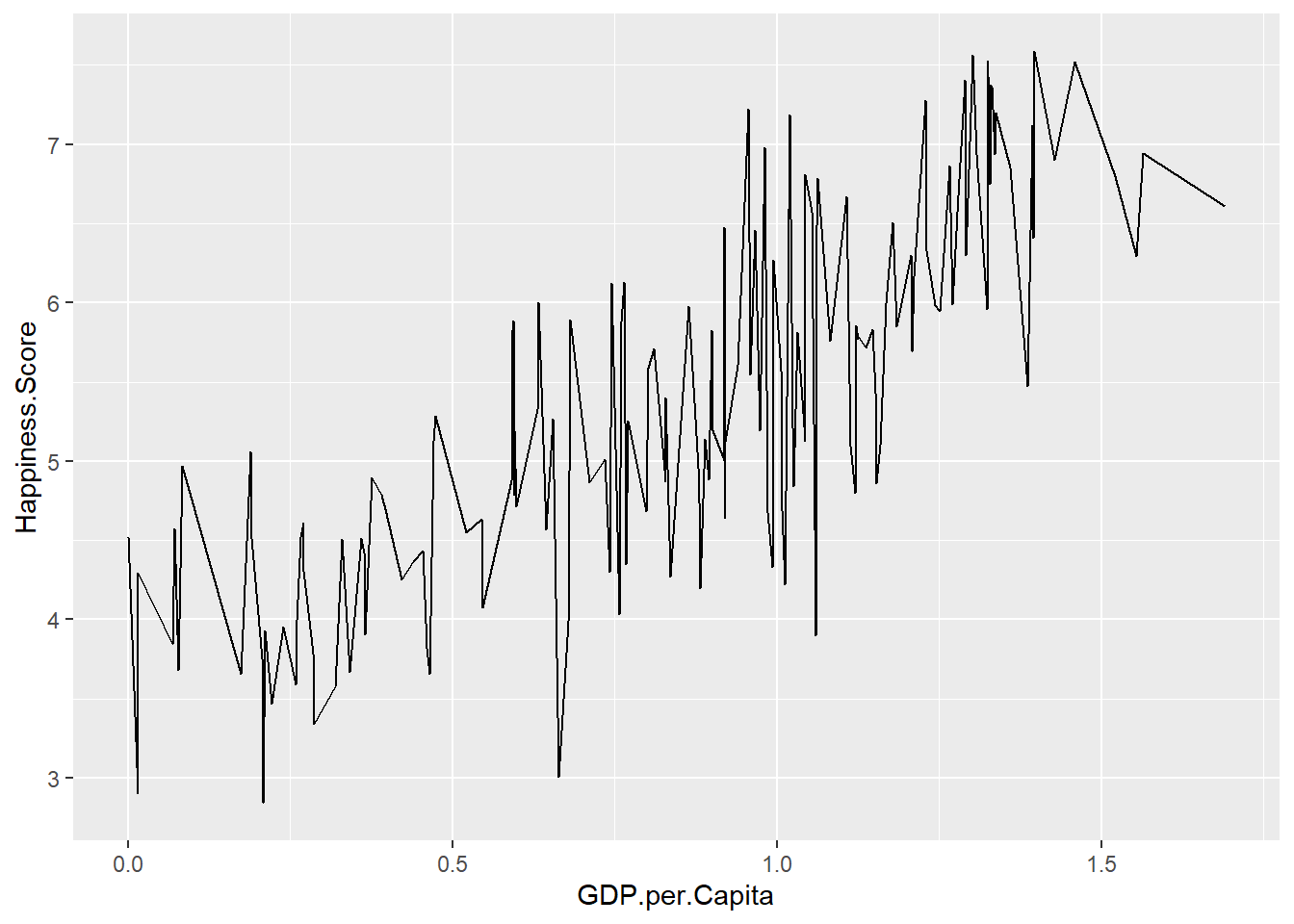The `geom_line` doesn’t make a lot of sense in this case but it is great for time series data.

We can add multiple `geom`s if we want. For instance, we can plot a smoothing trendline (`geom_smooth()`) overlaid on the scatterplot.

``````ggplot(data = WHR, aes(x = GDP.per.Capita, y = Happiness.Score)) +
geom_point() +
geom_smooth()``````
``## `geom_smooth()` using method = 'loess' and formula = 'y ~ x'``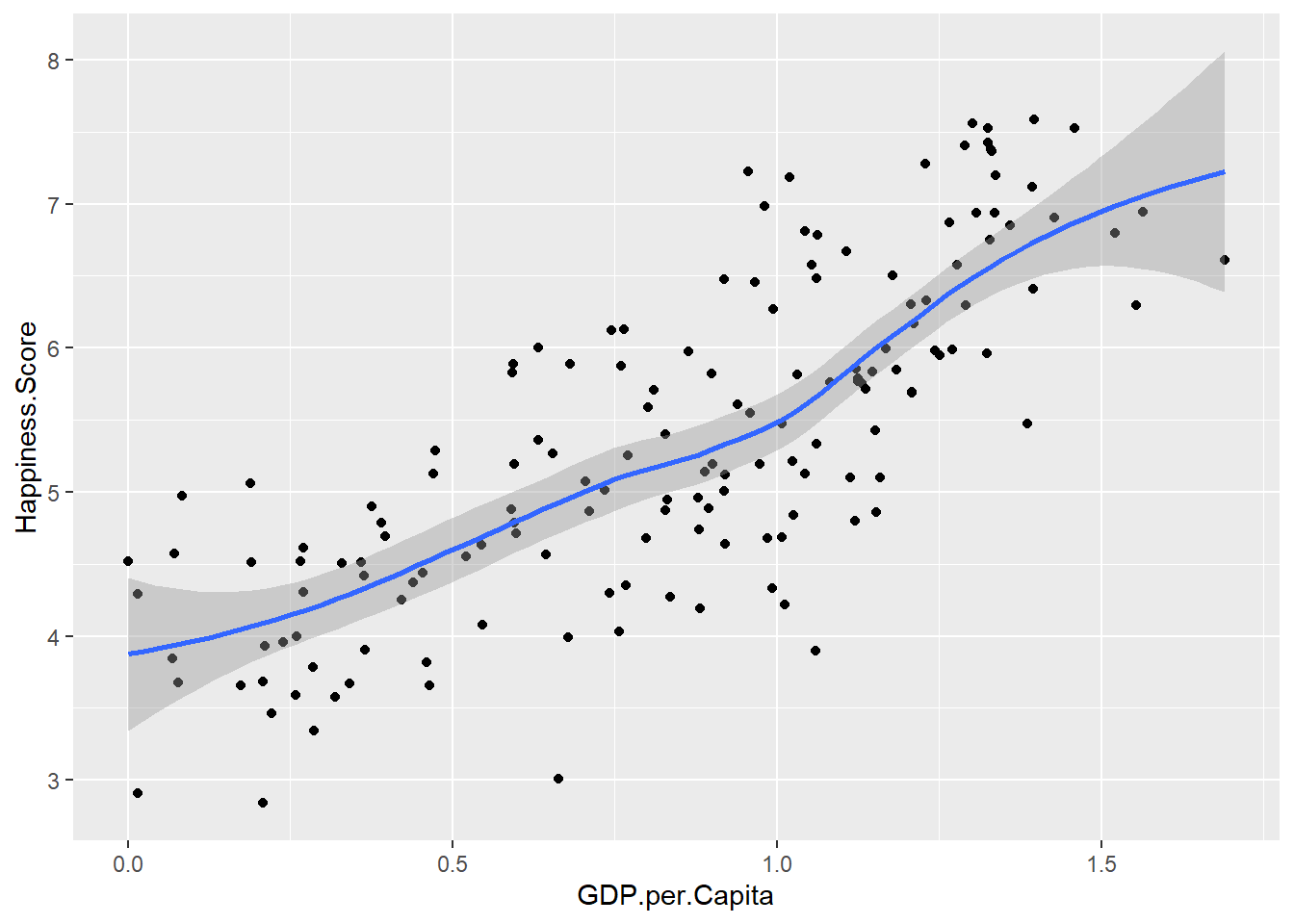Now we have a point plot with a trendline overlaid on top. The `geom`s are drawn in the order they are added to the plot.

Notice I have put each piece on its own line. This makes the code much easier to read especially if you are making a complex plot with dozens of lines of code.

By default, the `geom_smooth()` plots a locally weighted regression with standard error as a shaded area. We can change the type of trend to a linear trend by specifying `method = lm` as an argument, and get rid of the standard error shading by specifying `se = FALSE`.

``````ggplot(data = WHR, aes(x = GDP.per.Capita, y = Happiness.Score)) +
geom_point() +
geom_smooth(method = lm, se = FALSE)``````
``## `geom_smooth()` using formula = 'y ~ x'``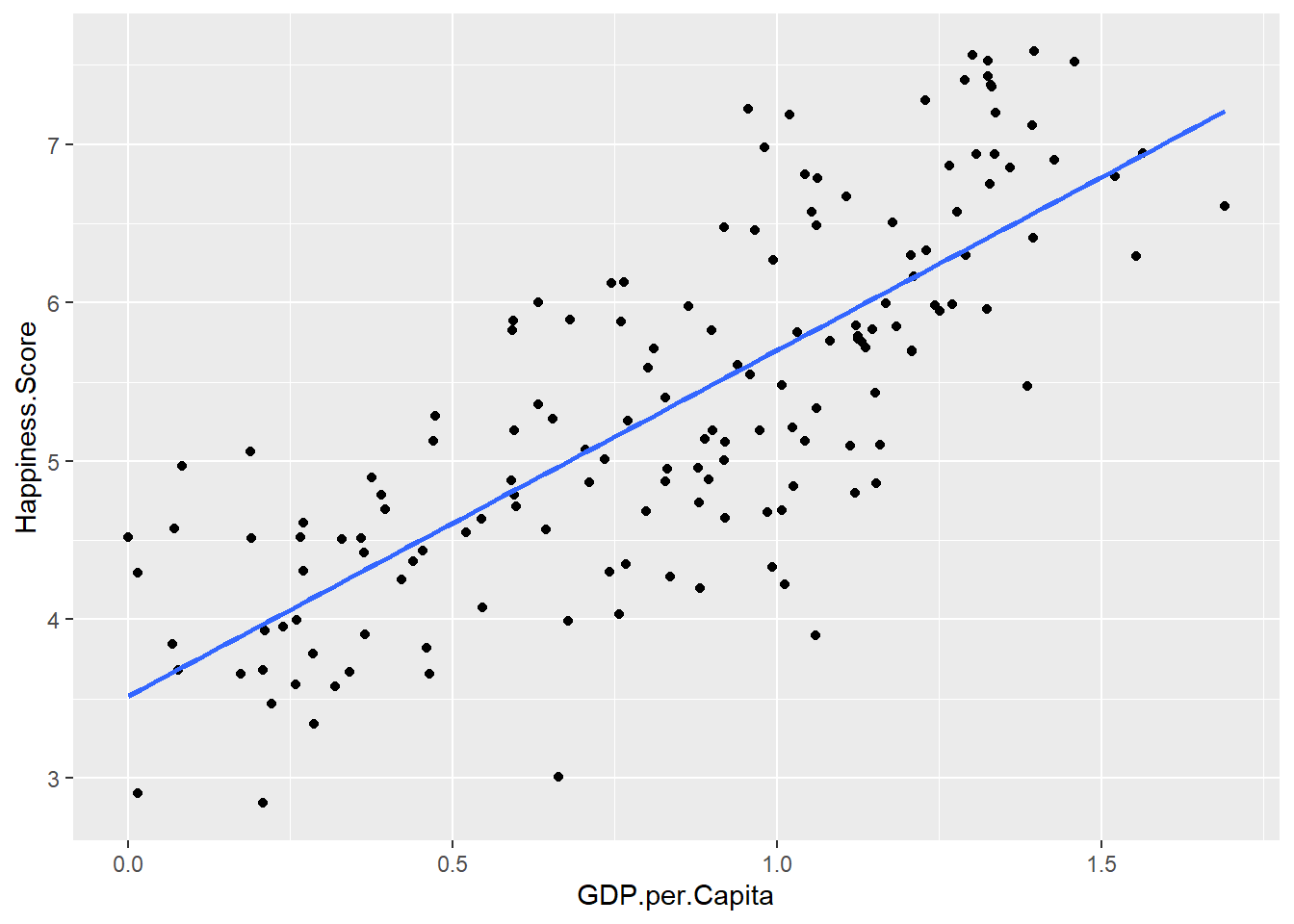### Modifying the plot: changing the aes

If we add to or change the `aes` arguments, we will modify or change what data are used to plot. For example let’s add a `color` aesthetic to the point plot to color each country’s point by continent.

``````ggplot(data = WHR, aes(x = GDP.per.Capita, y = Happiness.Score, color = Continent)) +
geom_point() +
geom_smooth(method = lm, se = FALSE)``````
``## `geom_smooth()` using formula = 'y ~ x'``